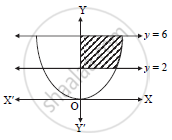HSC Arts 12th Board ExamMaharashtra State Board
Share

# Find the area of the region bounded by the curve x^2 = 16y, lines y = 2, y = 6 and Y-axis lying in the first quadrant. - HSC Arts 12th Board Exam - Mathematics and Statistics

ConceptArea of the Region Bounded by a Curve and a Line

#### Question

Find the area of the region bounded by the curve x2 = 16y, lines y = 2, y = 6 and Y-axis lying in the first quadrant.

#### Solutiony varies from y = 2 to y = 6.
Equation of parabola x2 = 16y

x=4sqrty

Required area = int_a^bxdy

=int_2^64sqrtydy

=4[y^(1/2)/(3/2)]_2^6

=4xx2/3[(6)^(3/2)-2^(3/2)]

=8/3[6^(3/2)-2^(3/2)]sq. units

Is there an error in this question or solution?

#### APPEARS IN

2016-2017 (July) (with solutions)
Question 4.2.4 | 2.00 marks

#### Video TutorialsVIEW ALL 

Solution Find the area of the region bounded by the curve x^2 = 16y, lines y = 2, y = 6 and Y-axis lying in the first quadrant. Concept: Area of the Region Bounded by a Curve and a Line.
S